# UNITS MASTERInnovative Unit Conversion Calculator

Work smarter and get instant results

Need fast, easy and accurate unit calculator and unit converter?
UNITS MASTER is a smart and innovative solution for unit calculation and conversion that saves your time and helps you avoid calculation mistakes. Due to the built-in intelligent data analyzing algorithm you may not only perform complex calculations but also easily adapt the app to your needs.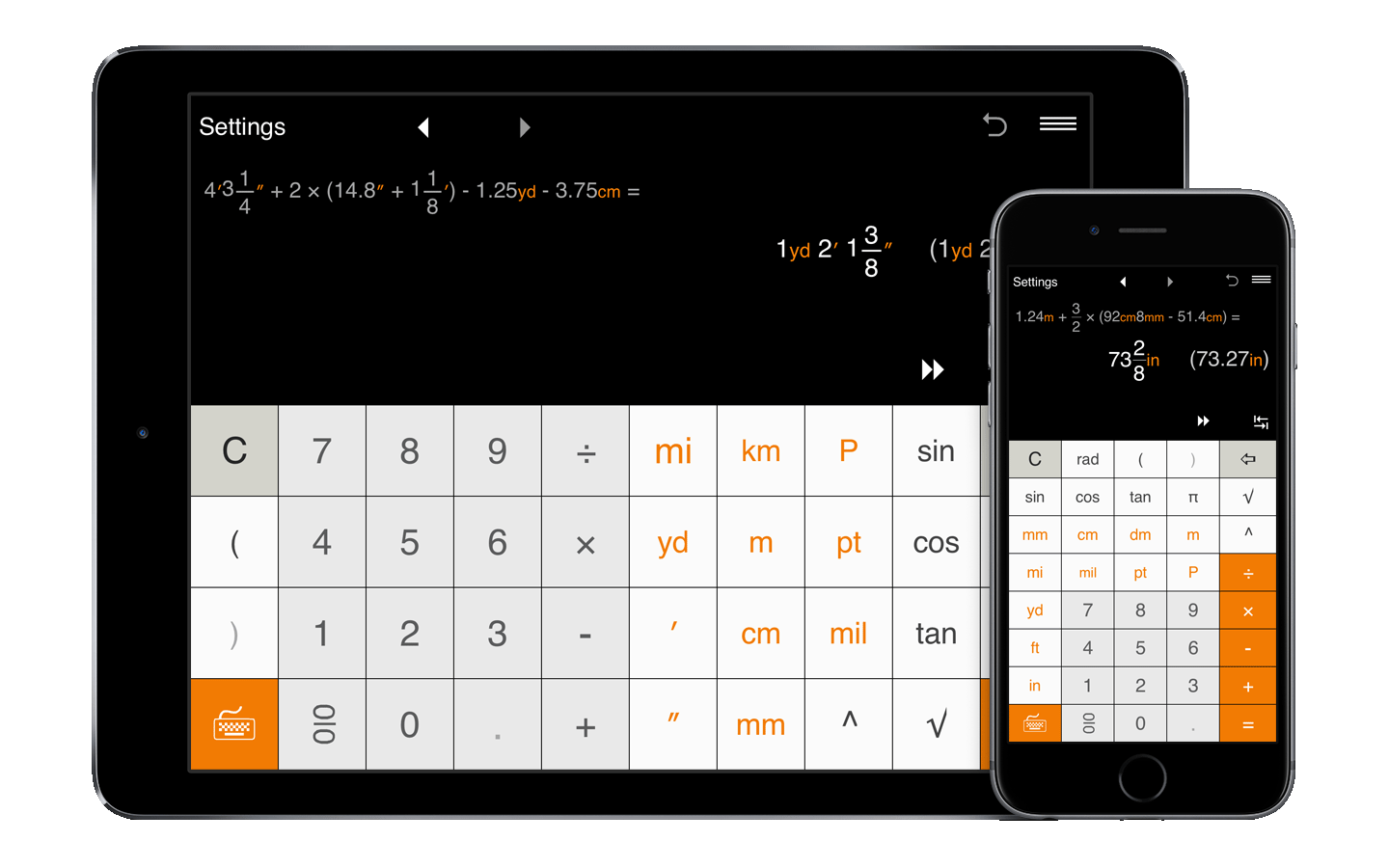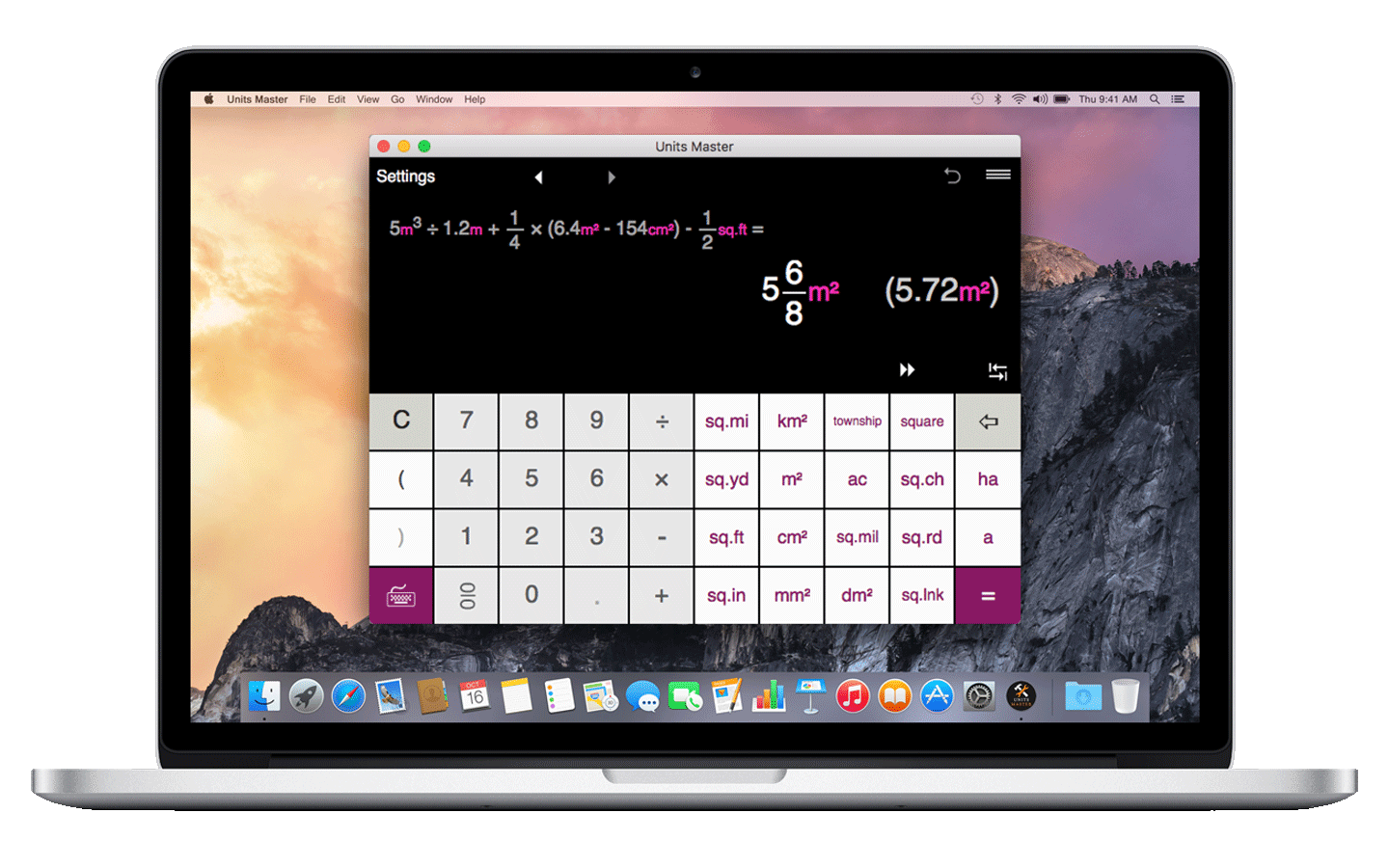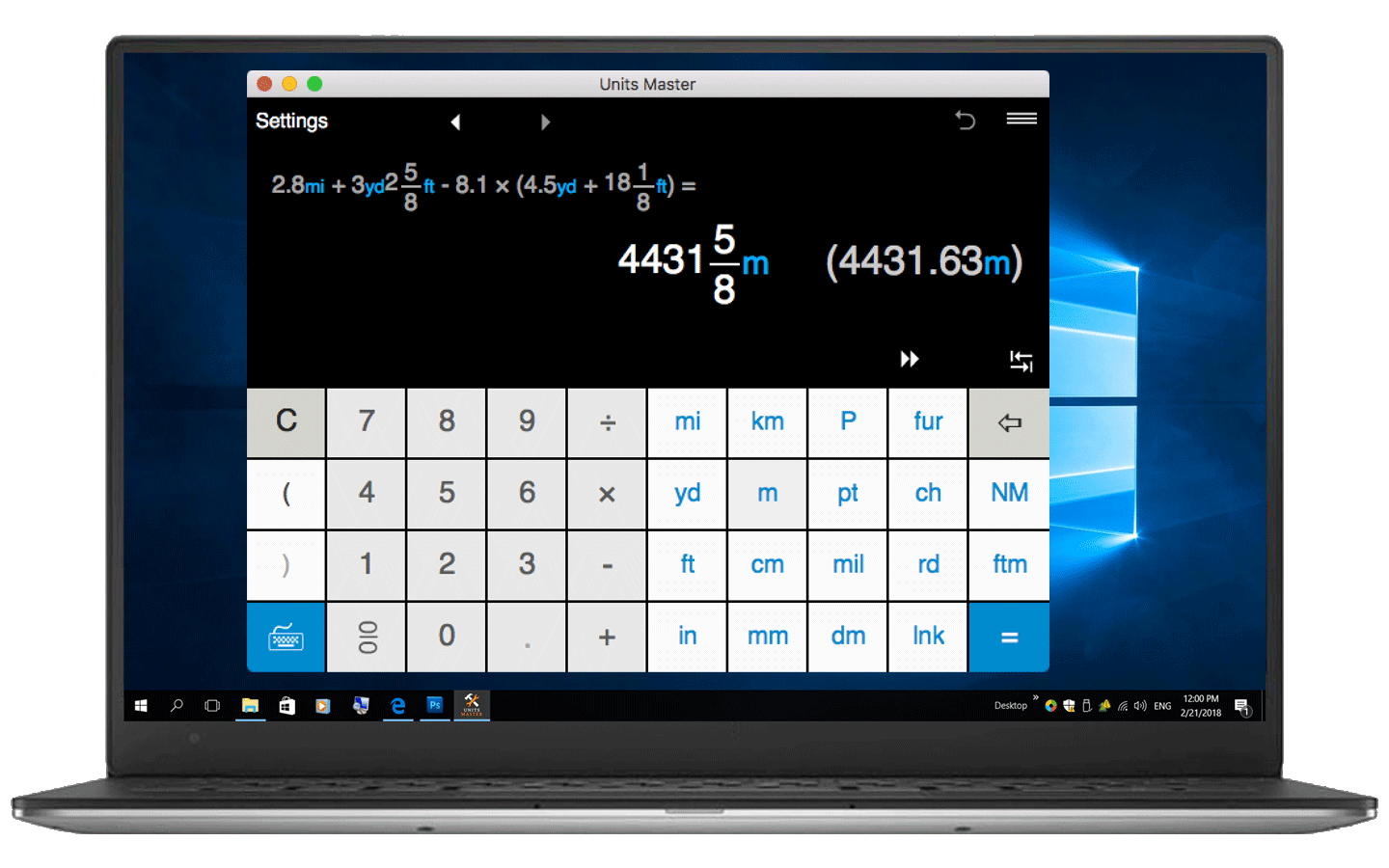Perfect for engineering, construction, building, scientific, technical and mathematical calculations with units, dimensional math and analysis or just unit conversion.

## Solve unit calculations and conversions on your smartphone, tablet or computer

### Full-featured unit calculator and converter

The Units Master App is a full-featured calculator and converter with clean interface and powerful solutions for calculating in fractional a decimal units in both US/imperial and metric systems.

### Quick to adjust and easy to use

The app provides simple input for units and fraction/decimal math, supports a natural display for fractions and shows both the expression and the result so you can easily check the expression and quickly identify and fix any input errors.

### Everyday and advanced unit calculations

Ideal for engineers, contractors, builders, architects, carpenters, designers, scientists, students and anyone who needs to perform unit conversion and calculation on a daily basis.

### Tailor the app to your needs

With Units Master, you may easily get the answer in desired unit(s) in fractional and decimal forms, round results to usable fractions, choose the default output format and more.

## Convert and calculate units of measure easily. Eliminate calculation errors. Increase productivity.

Units Master is both a unit calculator and a unit converter that supports up to 36 length and area units. The app is a must-have tool for everyone who needs to perform fast, accurate and efficient calculation with US standard, metric, and imperial units. Thousands of constructors, engineers, DIYs, plumbers, students, teachers, scientists, and travelers use Units Master every day to solve various unit problems from converting units within the same or between different measurement systems to performing advanced calculations. The tool would be also extremely useful for students who need to quickly check results while mastering their dimensional analysis skills.

Units Master provides a reliable and time-saving solution that allows users to improve productivity by streamlining both unit conversion and unit calculation. The app also helps users reduce errors and ensure accuracy of their results for better decision-making. Units Master can handle a wide range of math operations, including basic operations such as addition, subtraction, multiplication, and division, as well as more advanced ones such as square root, exponential and trigonometric calculations for both decimal and fractional values. Additionally, the app can store and recall previous calculations for easy reference.
Units Master is a platform-agnostic application which is available in App Store, Google Play Store, and Windows Store. You can use Units Master from anywhere and anytime on all devices including smartphones, tablets, and laptops.

Get the app now and make your life easier!
Unit conversion and calculation made easy!

## Units calculation

• Instantly calculate using 36 built-in standard (US) and metric length, distance and area units.
• Intuitive interface for units input.
• Calculate in fractions and decimals.
• Calculate linear and square measurements in metric units.
• Calculate linear and square measurements in US/imperial units, including feet-inch-fractions, inch fractions, decimal feet, decimal inches and more.
• Perform calculations when metric and US units are mixed.
• Convert results between different units with one click.
• Get the answer in desired unit(s) in a fractional and decimal form.
• The app displays both the expression and the result at the same time.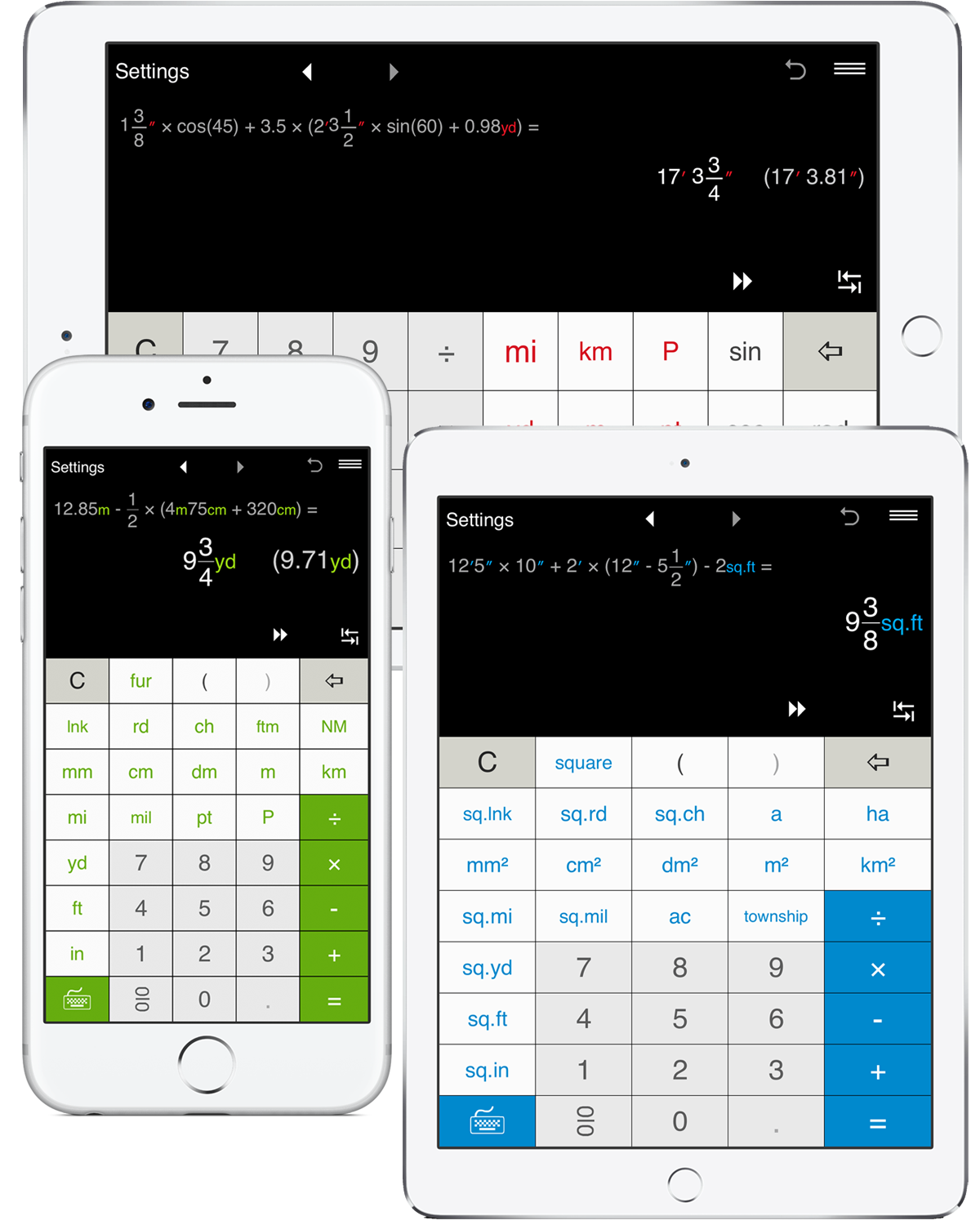## Professional unit conversion calculator

• More than 1000 conversion combinations.
• Convert between fractions and decimals:
- decimal to fraction conversion
- fraction to decimal conversion
• Convert between US, Imperial and metric length and area units, including commonly used inches/centimeters/millimeters, feet/yards/meters, square inches/square feet/m² and other length and area units conversions.
• Convert between units in the same system:
- conversion between metric units
- conversion between US units
• Fractional and decimal unit conversion, including foot-inch-fraction to decimal format conversion.
• One-click result conversion by clicking the unit button you want the result value converted to.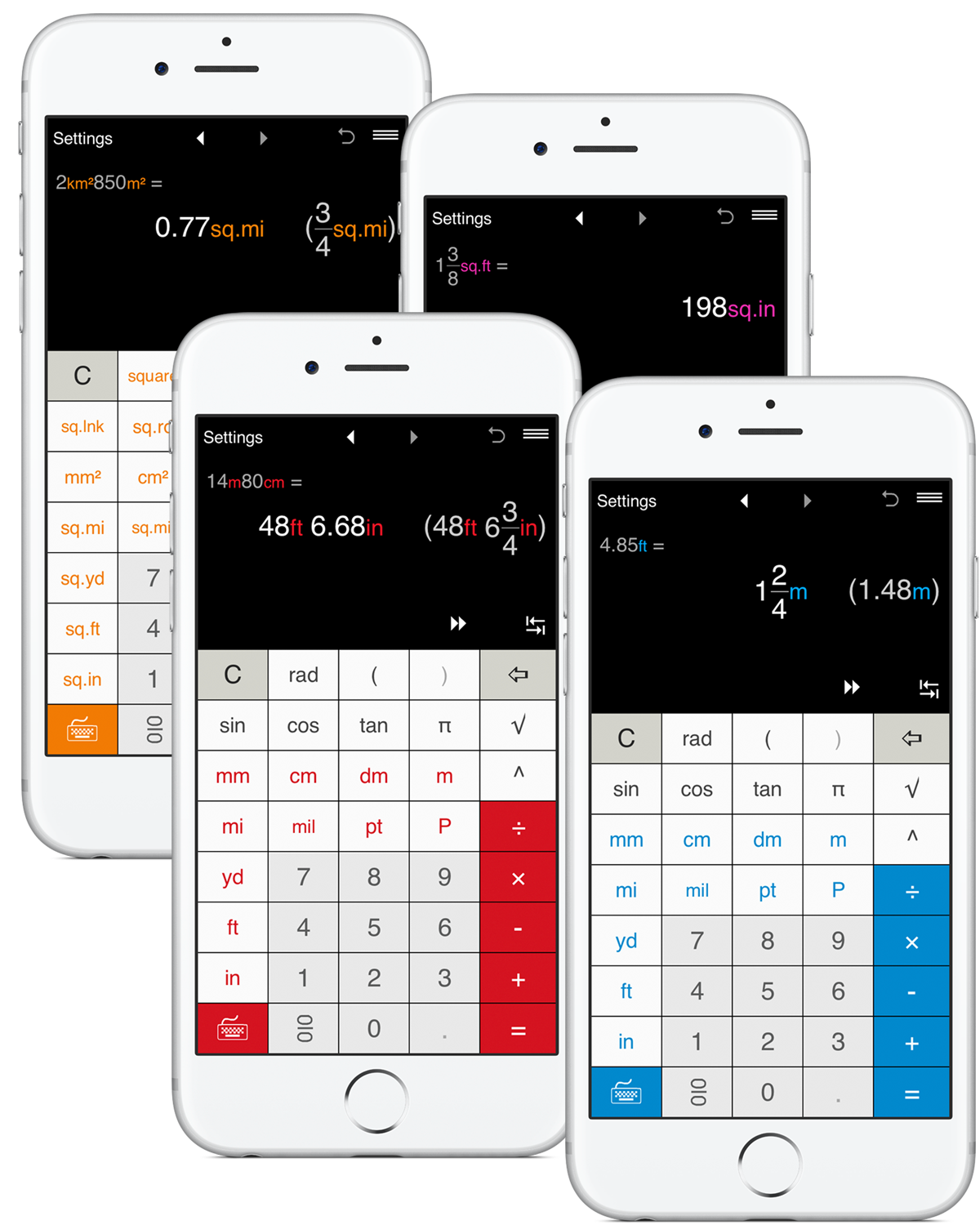## Works as a standard calculator

• Addition, subtraction, multiplication and division.
• Calculations with parentheses, including nested parentheses.
• Fraction and decimal math.
• Powers, trigonometric functions.
• Intuitive fraction input.
• Constants: π (pi).
• Trigonometric calculations in Degrees and Radians.
• Square Root.
• Fraction to decimal and decimal to fraction conversion.
• Reduce fractions and mixed numbers.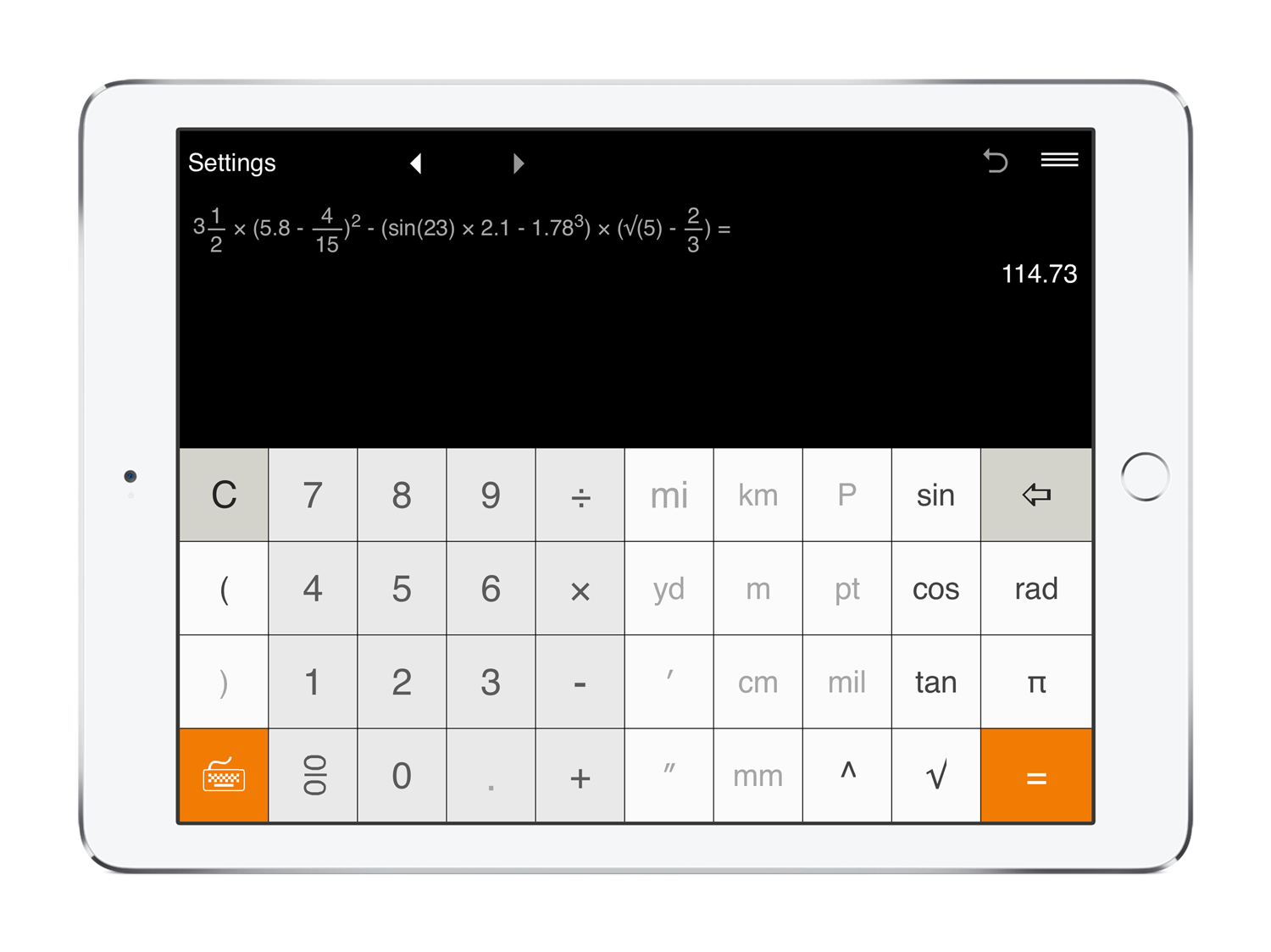## Settings

• Three output modes for results: Automatic, US (imperial) and Metric.
• Round results:
- Decimal numbers can be rounded to 0-8 of significant figures. You can set up the app to round decimals to tenths, hundredths, etc. By default, the result is rounded to 10ths.
- For fractions, you may round the results to the nearest 1/2, 1/4, 1/8, 1/12, 1/16, 1/32, 1/64, 1/128, 1/256. If you do not need to round fractions, just select "-".
• 7 color themes.
• Use the app in either portrait or landscape mode.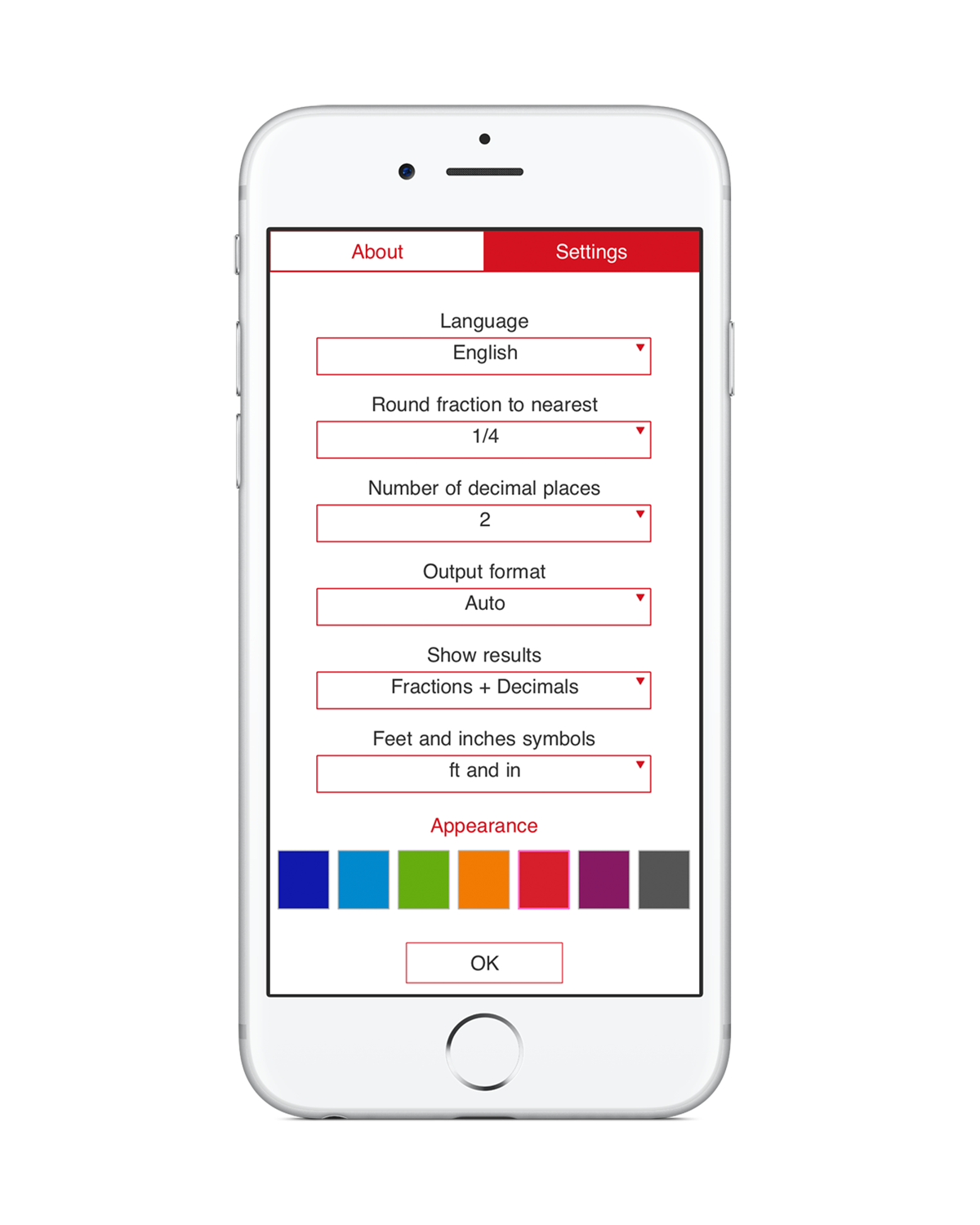## How you can benefit from the Units Master app

### Save Time and Reduce the Risk of Human Errors

If you’ve ever had to perform calculation involving inches and feet or conversion from yards to meters or from square kilometers to square miles, then you know that it’s not so easy and time consuming. First, you need to know the conversion factors and formulas. And even if you remember them or search for them, it takes a significant amount of time to complete the task. The problem becomes more complex if you need to operate with fractional values or even with a mixture of fractions and decimals. In this case you additionally need to care about fraction and decimal math.

One of the key advantages of using Units Master is the ability to save time and ensure reliable results by reducing the risk of inaccuracies that can occur while manual calculations. With the Units Master calculator, you can perform precise calculation and solve problems that would take hours to complete manually, in seconds.

### End-to-end solution for calculations of any complexity

While many online tools or traditional calculators support most frequent scenarios of unit calculation and conversion, they usually can’t solve complex problems. And, at the end, you still need to finalize the calculation on your own.

Solving problems involving the calculation and conversion of units of measurement is not a challenging task anymore. Units Master is designed and developed to help you with various types of calculations with units – from the problems when you need to add, subtract, multiply, or divide various units to situations when you need to calculate more complex expressions involving various combinations of supported units; fractions, decimals, and mixed numbers; brackets, trigonometric functions and more. The app uses a data analyzing algorithm, which supports intelligent input of complex expressions involving SI (or metric) units, US customary units and imperial units in both decimal and fractional formats. Equipped with all these options, Units Master saves you significant amount of time by performing complex calculations with length, distance, and area units instantly and accurately. Learn more about the problems you can solve using the app.

### Special Functions• Intuitive interface for fraction input.• Switch between the keyboards.• Convert results between standard and metric systems.• Convert results between units within the same system.• Back and Forward buttons to find and recall recent calculations.• 'Undo' for the Clear command.• History Tape to view and share calculations.• Share calculation results via email.

When it comes to unit conversion, you can search for an online tool that can help you with or use a scientific calculator with a built-in unit conversion functionality. While this could be an easy solution if you want to convert between units such as inches and millimeters or square feet and square centimeters, it may be much more difficult to find a tool that addresses niche needs and helps you convert between less commonly used units as points, links, mils, furlongs, square rods, square chains etc.

Supporting 18 length and 18 area (or squared) units, Units Master will take care of more than 1000 conversions. So, in addition to common conversions between millimeters, centimeters, meters, kilometers, inches, feet, yards and miles, the application will help you convert mils to mm, feet to chains, points to inches, millimeters to picas, links to feet, meters to rods, furlongs to miles, fathoms to meters and more. You can also easily switch between square inches, square feet, square yards, square miles, square millimeters, square centimeters, square decimeters, square meters, square kilometers, acres, hectares as well as more specialized units as square mils, square links, squares, square rods, square chains, ares and townships. The full list of supported units.

The app allows to convert within the US customary, imperial and metric systems of measurement or effortlessly switch between them. And the best thing that differs Units Master from many unit converters available on the market, is the capability to convert mixed units. For example, you can convert 1yd2ft3in, 1/5ft2in, 8km238m, 0.5m8cm9mm etc. And while doing this, you can easily choose to display the result in any supported unit or even in mixed units. You may choose to convert 3m95cm in feet-inches or yard-feet-inches.

### Calculate and convert units with ease

The main purpose of Units Master is to streamline unit calculation and conversion for whatever purpose you need. Despite many advanced functions, the app has clean user interface and has been carefully prepared in a way that makes a learning curve as short as possible. For more information visit the Units Master FAQ and Help Section.

Users are not all the same. They have different needs and preferences. That’s why Units Master offers lots of customization options so you can easily choose input and output formats, formulas, rounding options, color schemes, and language of the app that work the best for you allowing you to get the best outcome. Explore all the customization options to get the most out of Units Master.

CS - Čeština

DE - Deutsch

DK - Dansk

EL - Ελληνικά

EN - English

ES - Español

FI - Suomi

FR - Français

IT - Italiano
JP - 日本語

KR - 한국어

NL - Nederlands

NO - Norsk (bokmål)

PT - Português

RU - Русский

SV - Svenska

TR - Türkçe

ZH - 中文